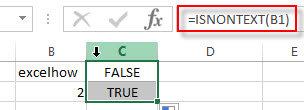# Excel ISNONTEXT Function

This post will guide you how to use Excel ISNONTEXT function with syntax and examples in Microsoft excel.

### Description

The Excel ISNONTEXT function used to check if a value is text. If so, returns FALSE; if the text is not text, the function will return TRUE. So you can use the ISNONTEXT function to check for a value that is not text in Excel.

The ISNONTEXT function is a build-in function in Microsoft Excel and it is categorized as an Information Function.

The ISNONTEXT function is available in Excel 2016, Excel 2013, Excel 2010, Excel 2007, Excel 2003, Excel XP, Excel 2000, Excel 2011 for Mac.

### Syntax

The syntax of the ISNONTEXT function is as below:

`=ISNONTEXT (value)`

Where the ISNONTEXT function argument is:

• Value -This is a required argument. The value that you want to test.

### Excel ISNONTEXT Function Example

The below examples will show you how to use Excel ISNONTEXT Function to check if a value is not text. And the ISNONTEXT function will return TRUE when value is not a text string.

#1 checks whether the value in Cell B1 is not a text string, using the following formula:

= ISNONTEXT (B1)### Related Functions

• Excel ISNUMBER Function
The Excel ISNUMBER function returns TRUE if the value in a cell is a numeric value, otherwise it will return FALSE.The syntax of the ISNUMBER function is as below:=ISNUMBER (value)
• Excel ISERR Function
The Excel ISERR function returns TRUE if the value is any error value except #N/A.The syntax of the ISERR function is as below:= ISERR (value)…
• Excel ISError Function
The Excel ISERROR function used to check for any error type that excel generates and it returns TRUE for any error type, such as: #N/A, #VALUE!,#REF!,#DIV0!, #NAME?, etc. The syntax of the ISERROR function is as below:= ISERROR (value)…
• Excel ISBlank Function
The Excel ISBLANK function returns TRUE if the value is blank or null.The syntax of the ISBLANK function is as below:=ISBLANK (value)…
• Excel ISNA Function
The Excel ISNA function used to check if a cell contains the #N/A error, if so, returns TRUE; otherwise, the ISNA function returns FALSE. The syntax of the ISNA function is as below:=ISNA(value)…
• Excel ISTEXT Function
The Excel ISTEXT function used to check if a value is text. If so, returns TRUE; if the text is not text, the function will return FALSE. The syntax of the ISTEXT function is as below:=ISTEXT(value)…
• Excel ISREF Function
The Excel ISREF function used to check if a value is a valid reference. If so, returns TRUE; if the value is not a reference, the function will return FALSE. The syntax of the ISREF function is as below:=ISREF(value)…